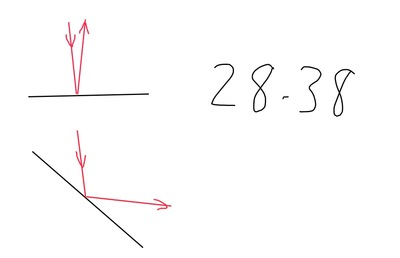## Conceptual Physics (12th Edition)

When the mirror is rotated by an angle X, its normal also rotates by an angle X. The angle that the incident light ray makes with the normal is the same as the angle that the reflected light ray makes with the normal, so the deviation between them grows by an amount 2X. In the diagram, the mirror is rotated by $45^{\circ}$, and the normal is rotated by the same amount. The reflected ray is rotated by $90^{\circ}$.